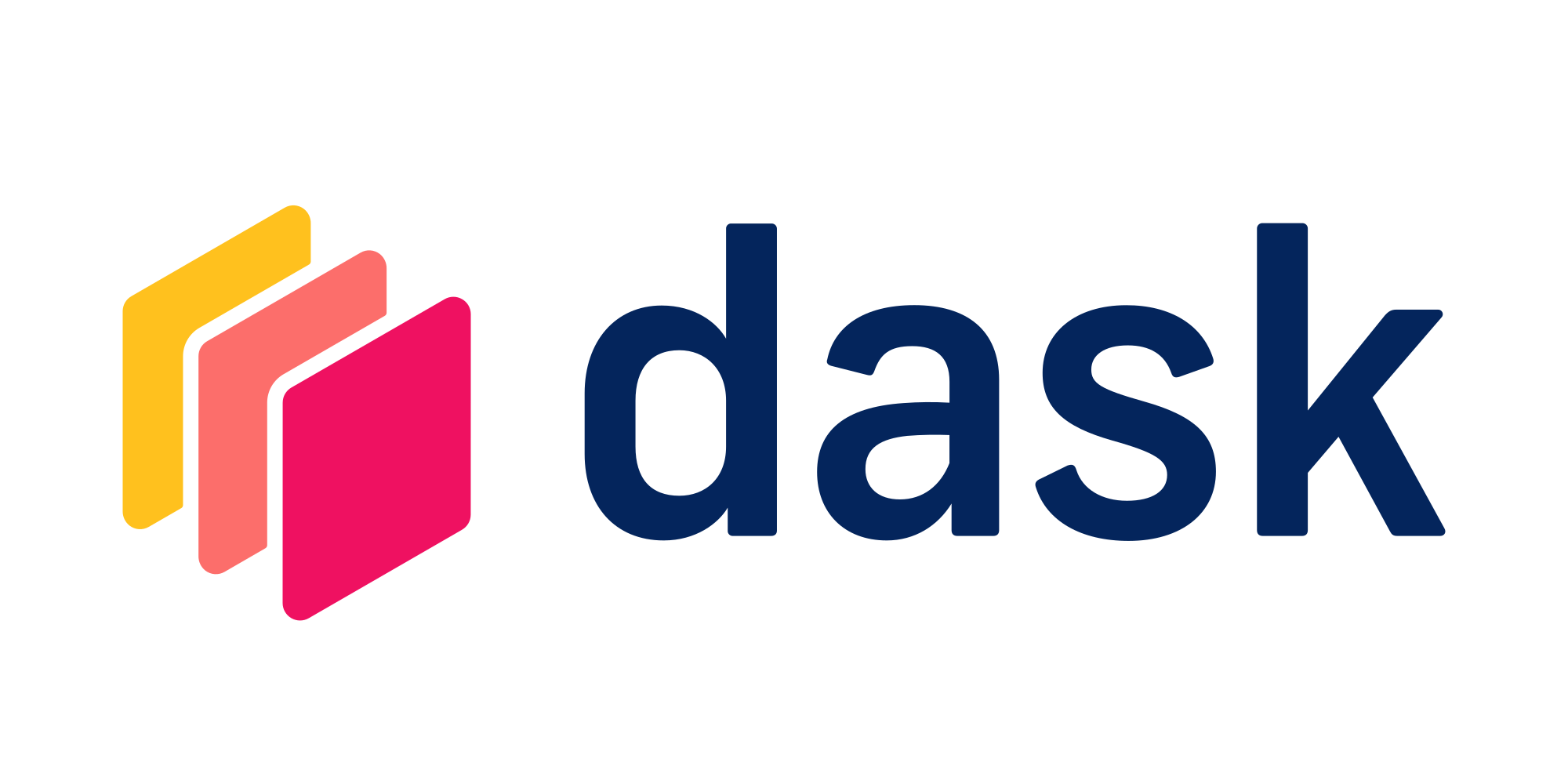Use Dask Arrays for distributing arrays across multiple machinesTry this example in seconds on Saturn Cloud

Dask arrays are similar to NumPy arrays, however they are distributed across a Dask cluster. They mimics the functionality of NumPy arrays using a distributed backend requiring minimal changes to your code. This example walks through using a Dask array on Saturn Cloud.

``````from dask_saturn import SaturnCluster

client = Client(SaturnCluster())
``````

After running the above command, it’s recommended that you check on the Saturn Cloud resource page that the Dask cluster as fully online before continuing. Alternatively, you can use the command `client.wait_for_workers(3)` to halt the notebook execution until all three of the workers are ready.

## Create a Dask Array from a NumPy Array

Function `from_array` allows us to create a Dask array from array like structures. The code below creates a Dask array out of NumPy array.

``````import numpy as np

y = da.from_array(
np.array([[1, 2, 3, 4], [5, 6, 7, 8], [9, 10, 11, 12], [13, 14, 15, 16]]), chunks=(2, 2)
)
``````

In code above, parameter `chunks` is telling us how to create blocks for this array. In this case we have created 4 blocks of equal size 2x2.

## Create a Dask Array directly

We can also create a Dask Array without using NumPy as an intermediary. In the code below a Dask Array is being created with all ones.

``````z = da.ones((4, 4), chunks=(2, 2))
``````

Dask Arrays can be converted to and from Dask DataFrames. Here we are using method `to_dask_array`, to convert a Dask DataFrame to a Dask Array.

``````import pandas as pd

df = pd.DataFrame([{"x": 1, "y": 2, "z": 3}, {"x": 4, "y": 5, "z": 6}])
df1 = dd.from_pandas(df, npartitions=1)
x.compute()
``````

## Example: Concatenating the array, slicing the array and finding mean of that sliced portion

In code below we take two of the arrays created above and concatenate them. The result is sliced and the mean is computed for that portion. Due to Dask’s lazy evaluation, these arrays will not be computed until we explicitly ask Dask to perform the computation. Hence in the end of all the functions we add `compute()`.

``````da.concatenate([y, z], axis=0)[1:].mean().compute()
``````

## Best Practices:

1. If possible, use NumPy arrays for as long your computations are fast enough and you data fits into a single machine.
2. Choose the chunk dimensions which are small enough to fit in memory but are big enough to avoid large overheads during operations.
3. Choose the shape of chunk wisely–if you are creating a Dask array out of HDF file which has chunks of dimensions 64x32 then you should create Dask array chunks in multiples of those dimensions.

For more details on using Dask Arrays, see the official Dask Array Documentation.

For more tips and tricks of using Dask check out the Saturn Cloud Blog.

``````from dask_saturn import SaturnCluster

client = Client(SaturnCluster())

import numpy as np

y = da.from_array(
np.array([[1, 2, 3, 4], [5, 6, 7, 8], [9, 10, 11, 12], [13, 14, 15, 16]]), chunks=(2, 2)
)

z = da.ones((4, 4), chunks=(2, 2))

import pandas as pd